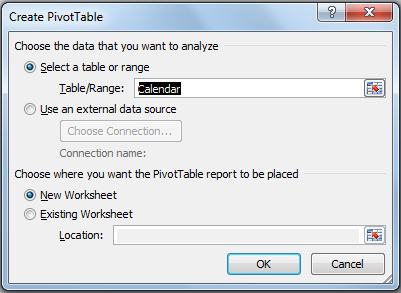#### How to Use Cubemember Function

CUBEMEMBER is a function that returns a member or tuple from the cube, it is used to validate that the member or tuple exists in the cube. If the value doesn’t exist, the formula will not work.

It allows data from a data model to be shown. Regular Excel formulas are not able to do that but luckily we have cube functions, including CUBEMEMBER. They are able to interact with the data model that is in PowerPivot.

## Cubemember function arguments

This function has three arguments:

Connection: This one is mandatory, it is a text string of the name of the connection to the cube.

Member_expression: This one is also required, it is a text string of a multidimensional expression that transforms into a unique member in the cube. It can also be a tuple, that is specified as a cell range or an array constant.

Caption: This argument is optional, it is a text string that is displayed in the cell instead of the caption, only if one is defined from the cube. The caption is used for the one last member in the tuple when it is returned.

## How to use cubemember function?

To use this function we first have to do some things before that. You have to make a PivotTable using the data model that you also created.

1. Go to the “Insert” tab,
2. Choose Pivot Table,
3. Select this Workbook’s Data Model and click Ok.After the last step you will get the option to select PivotTable fields. Select the right time interval and the value you are going to show, and you have your own little PivotTable. Let’s say for the purpose of this article that we have our PivotTable that shows the exact year and total sales.

Now you will want to convert the PivotTable to formulas.

2. Once you have that you should go to the ribbon and find the “PivotTable Analyze” section,
3. There you will see “OLAP Tools” and,
4. Then click “Convert to formulas”.Everything should look like the original PivotTable, the output is the same but the values are going to be shown in CUBEMEMBER formulas.

## Cubemember function examples

Here are few formulas from an example, they look like this:

=CUBEMEMBER(“ThisWorkbookDataModel”;”[Measures].[Total Sales]”)

=CUBEMEMBER(“ThisWorkbookDataModel”;”[Calendar].[Day Name].&”)

=CUBEMEMBER(“ThisWorkbookDataModel”; “[Calendar].[Day Name].[All]”;”Total Amount”)

The first one showcases the number of total sales that we got, while the last two show a period of time where we had a specific amount of sales.

CUBEMEMBER is there to verify the validity of written data from the data model. It usually shows column references and measures. Examples that we used were day names and total sales, but it can also show anything that is referenced in the PivotTable.

## Conclusion

The CUBEMEMBER function is pretty useful. It is simple to use and it can really help a lot.

All cube functions are good in general, they represent a link between the Data Model and the Excel Worksheet, you will surely need to use them at least once.